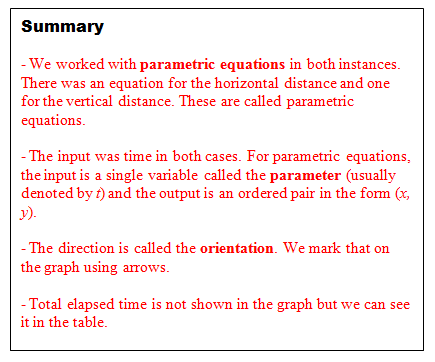# The Stolen Car and Keys: An Introduction to Parametric Equations

5 teachers like this lesson
Print Lesson

## Objective

SWBAT graph parametric equations.

#### Big Idea

A car and set of keys have been stolen - what does this have to do with parametric equations?

## Launch and Explore

25 minutes

The purpose of today’s lesson is to give students two contexts that will build conceptual understanding of parametric equations. I really want my students to understand that there is a single input (usually time) and an ordered pair output. I feel that this is a great lesson to build that knowledge. I adapted these tasks from similar ones I found in a College Preparatory Mathematics textbook.

I give students the task worksheet and I have them work on it with their table groups. It is quite lengthy so I usually give them 20 – 30 minutes. As they are working on it I will circulate around the room to make sure that they are on the right track and to look out for general trends. Here is a list of a few things that I will key in on:

1. Are students graphing correctly (e.g. not putting t on the x-axis)?
2. Are the scales of the graph appropriate and accurate?
3. What assumptions of the path of the car or the keys are students making?
4. Do students understand what the input and output are?

## Share

20 minutes

After students have had sufficient time to work we will start discussing as a class. I will choose a student to share graphs in both instances as our starting point. Then we will discuss the questions. In this document I added some notes (in red) to show what answers and ideas I want to bring out in class. If these ideas do not come out naturally by my students, I will ask questions to get us there.

For question #7, it can be really surprising that the speed of the wind has no affect on when the keys will hit the ground. It is counterintuitive for some students to think that the keys will hit the ground at 4 seconds regardless of how fast the wind is going. Mythbusters did an awesome demonstration to prove that this is true. I show this clip to my students to convince them.

## Summarize

5 minutes

To close the lesson, I introduce the vocabulary associated with parametric equations. Students will write this summary down in the box at the end of their worksheet. The important vocabulary is shown below.